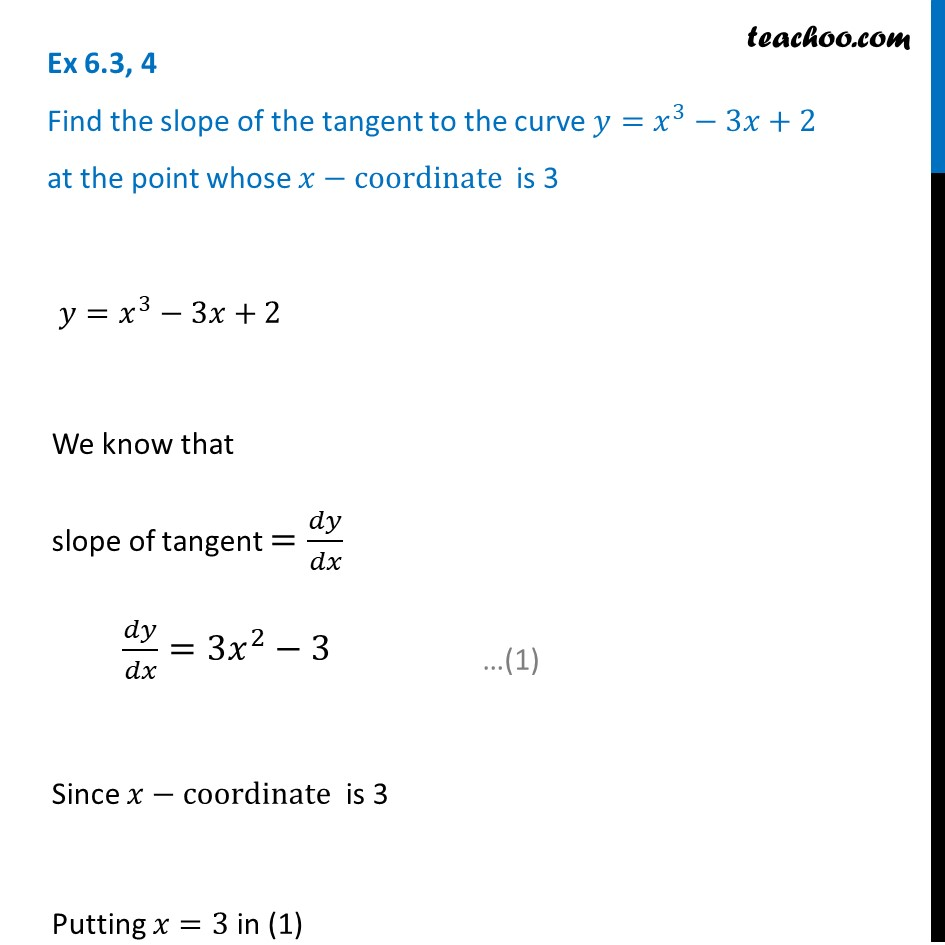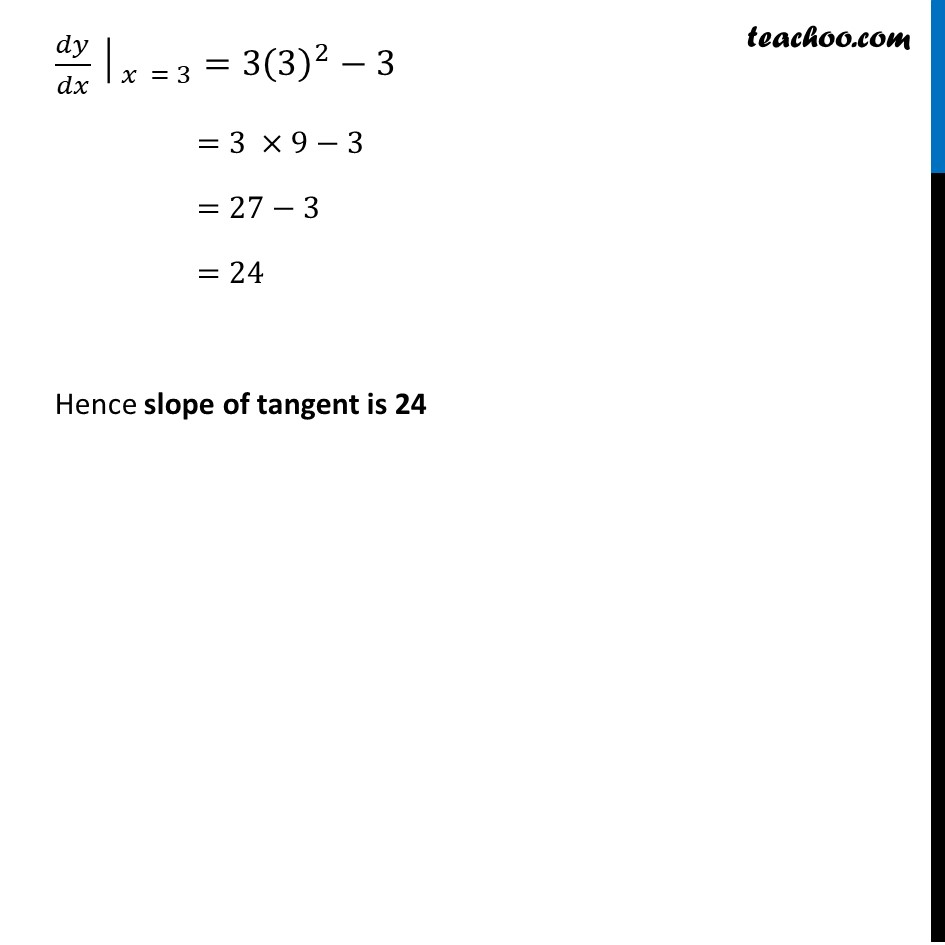Tangents and Normals (using Differentiation)

Chapter 6 Class 12 Application of Derivatives
Serial order wiseLearn in your speed, with individual attention - Teachoo Maths 1-on-1 Class

### Transcript

Question 4 Find the slope of the tangent to the curve 𝑦=𝑥^3−3𝑥+2 at the point whose 𝑥−coordinate is 3 𝑦=𝑥^3−3𝑥+2 We know that slope of tangent =𝑑𝑦/𝑑𝑥 𝑑𝑦/𝑑𝑥=3𝑥^2−3 Since 𝑥−coordinate is 3 Putting 𝑥=3 in (1) 〖𝑑𝑦/𝑑𝑥│〗_(𝑥 = 3)=3(3)^2−3 =3 ×9−3 =27−3 =24 Hence slope of tangent is 24